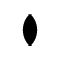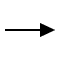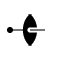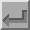Twofold Axis (2)The symbol above shows a twofold axis perpendicular to the plane of the screen, i.e parallel to Z. A twofold axis is equivalent to a rotation of 180° about a line. A twofold rotation about the c-axis, i.e. about the line 0,0,z, will have the corresponding symmetry operator -x,-y,z. This axis has the written symbol "2".The symbol shown above corresponds to a twofold axis, but now parallel to the Cartesian X direction. A twofold rotation about the x-axis will have the corresponding symmetry operator x,-y,-z. A fractional value next to the symbol indicates the height of the axis of rotation above the XY plane.In space group diagrams for cubic symmetry, twofold axes may appear inclined at an angle of 45° to the XY plane. The symbol for these is shown above, the dot indicating the exact position of intersection with the plane.Back
 © Copyright 1995-2006.  Birkbeck College, University of London. Author(s): Jeremy Karl Cockcroft